Next: Newton's 3rd Law Up: Newton's Laws Previous: Newton's 1st Law

## Newton's 2nd Law

The 2nd law of Newton describes what happens quantitatively when a force does act upon an object: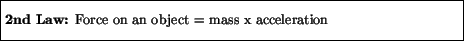We thus see that the acceleration of an object is always proportional to the force acting on it. Double the force, and the acceleration doubles; halve the force and the acceleration is cut in half. Also, since acceleration has a direction associated with it, so must the force.

Note that the mass of the object (also called the inertial mass) determines how much effect a given force has on that object. The greater the mass, the harder the object is to accelerate. This relation between force, mass, and acceleration agrees with our intuitive concept of what a force is: a given force will cause a small mass to accelerate more than a large mass, and for a given mass a larger force will cause a greater acceleration. From the 2nd law we can also see that the units of force must be (kg m/s2), which are given the special name of Newton (N). That is, 1 Newton = 1 kg m/s2 is the force required to accelerate a 1 kilogram object at a rate of 1 m/s2.

We note that the 1st law may in effect be considered as a special case of the 2nd law: when the force on an object is zero, the 2nd law states that the acceleration must be zero. This implies that the velocity doesn't change, which is simply the statement of the 1st law.

Newton's 2nd law is sometimes phrased in a different form. Since acceleration is the rate of change of velocity, we have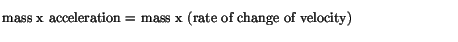which, if the mass doesn't change in time, can be written as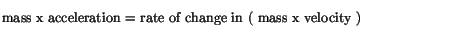One then defines the momentum of an object as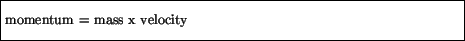Note that a large momentum can arise from a large mass and/or a large velocity: a train moving at 5 km/hr has a great deal more momentum than a ping pong ball moving at the same speed. In terms of momentum, Newton's 2nd law has the form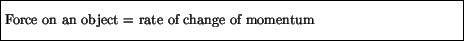Thus, a force changes the momentum of an object. A large force will change the momentum rapidly, whereas a small force will change the momentum slowly. Newton's 2nd law therefore agrees with our everyday experience with momentum: it takes a large force to affect a big change in momentum in a short period of time. A ping pong ball moving at 5 km/hr can be stopped quickly with a small force (you can catch it in your hand), whereas to stop a train moving at the same speed would either take a much larger force, or a longer time: it is in principle possible for an ordinary person to stop a moving freight train with his or her bare hands. Assuming the person did not drop from exhaustion first, it would just take a very long time.Next: Newton's 3rd Law Up: Newton's Laws Previous: Newton's 1st Law
modtech@theory.uwinnipeg.ca
1999-09-29